Open in App
Not now

# Straight Line Method of Charging Depreciation

• Last Updated : 22 Aug, 2022

Businesses choose different methods for calculating depreciation according to their need. One of the most prominent methods for calculating depreciation is the Straight Line Method. Under this method of charging depreciation, the amount charged as depreciation for any asset is fixed and equal for every year. The amount of depreciation is deducted from the original cost of an asset and charged on the debit side of the Profit and Loss A/c as a loss. The concerned asset is depreciated with an equal amount every year until the book value of the asset becomes equal to the scrap value of the asset. It is also called the ‘Equal Installment Method’ or ‘Fixed Installment Method’.

#### Merits of Straight Line Method:

1. Simplicity: The calculation of depreciation, under this method is not much complicated. The simplicity of the method is also a reason behind its popularity.

2. Equality of Depreciation Burden: In this method, the depreciation charged for every year is equal. Hence, the amount transferred to Profit and Loss A/c for each year is equal and the burden of depreciation on the Net Profit of the company for every year will be the same.

3. Assets can be Completely Written off: The book value of the asset under this method can be depreciated until it becomes zero or equal to the scrap value of the asset. This helps companies in writing off an asset completely.

4. Knowledge of Original Cost and Up-to-date Depreciation: Under this method, the original cost of the asset is shown in the balance sheet along with the deduction of the depreciation for the year. This helps businesses in keeping up with the information regarding depreciation for the year and the actual cost.

#### Formula for Calculating Depreciation:

1. When Scrap Value is Given:2. When Rate of Depreciation is Given:#### Journal Entries:

1. At the time of Purchase of Fixed Assets: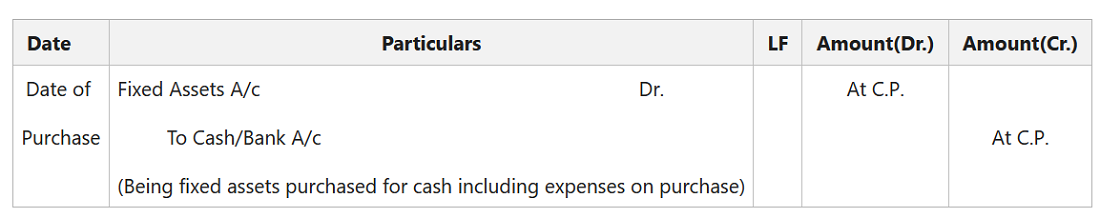2. At the End of Each Accounting Period:

(i) For the Depreciation charged on Fixed Assets:

(ii) For Transferring Amount of Depreciation to Profit & Loss A/c: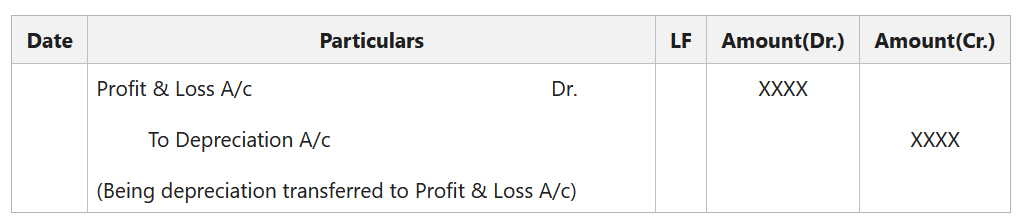3. At the Time of Sale of Fixed Assets:

(i) For the Depreciation charged on Selling Assets (Up to the Date of Sale):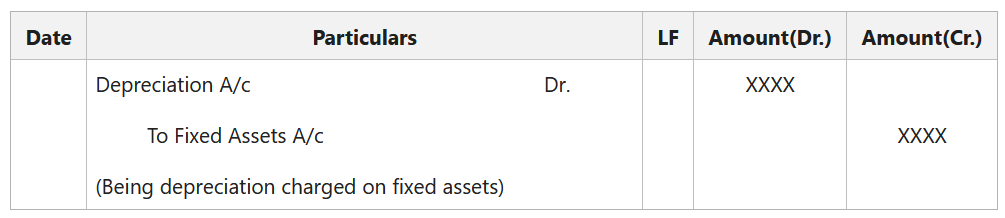(ii) For the Sale of Fixed Assets: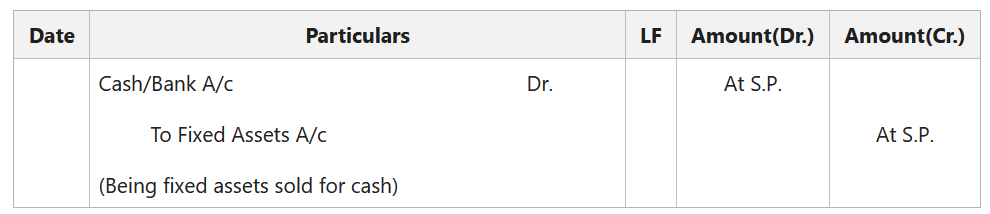(iii) For the Profit/Loss on Sale of Fixed Assets:

• For the Profit on Sale of Fixed Assets (If Sale Price is More than the Book Value):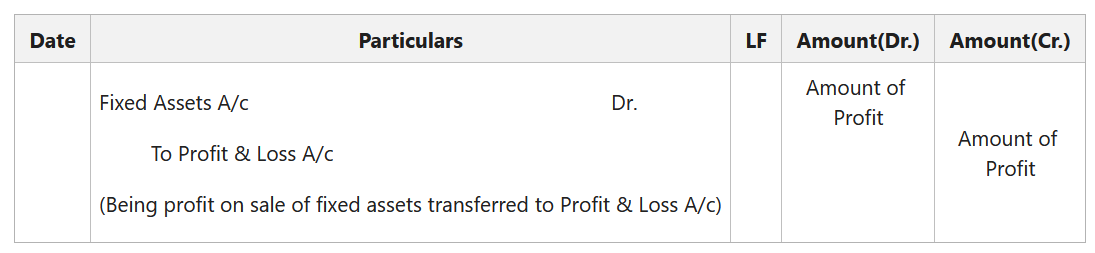• For the Loss on Sale of Fixed Assets (If Sale Price is Less than the Book Value):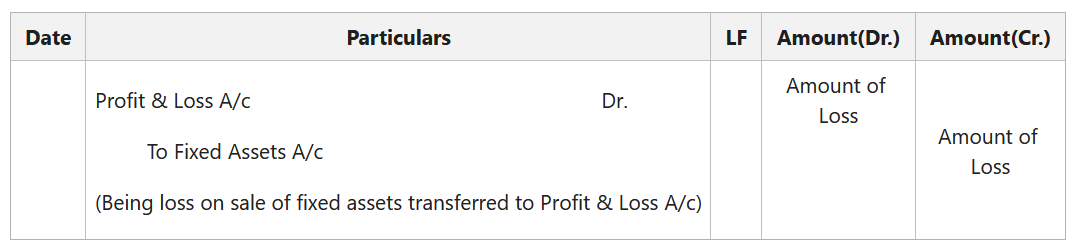Illustration:

A machine was purchased on 1st April 2019 for ₹39,400 and ₹600 was spent on its installation. On 1st October in the same year, additional machinery costing ₹20,000 was acquired. On 1st October 2021, the machinery purchased on 1st April 2019 was sold for ₹22,000 and on the same date, new machinery was purchased for ₹10,000. Depreciation is provided annually on 31st March @ 10% per annum on the original cost of the asset. Pass the Journal Entries and prepare Machinery Account from 2019-2021.

Solution: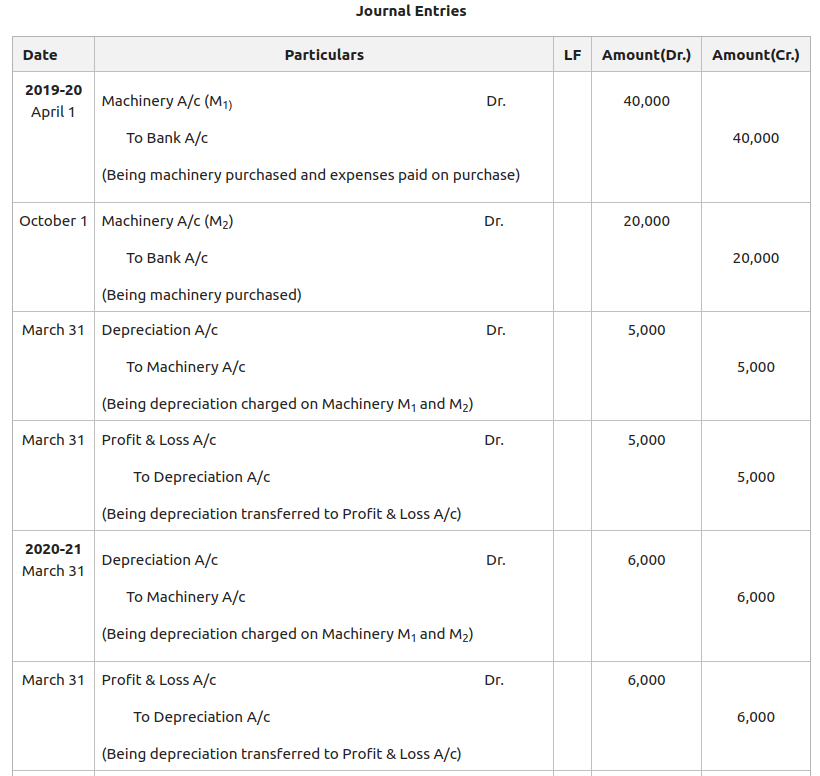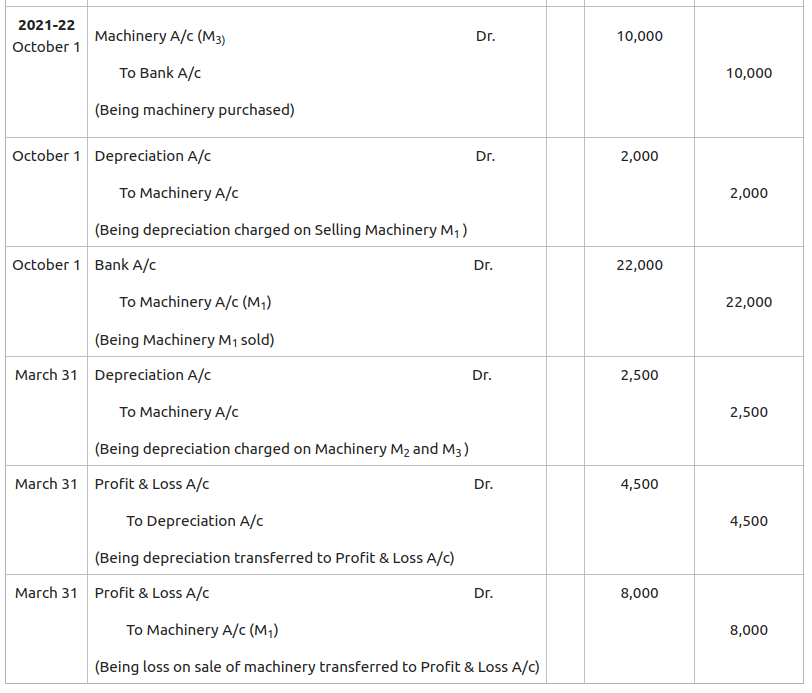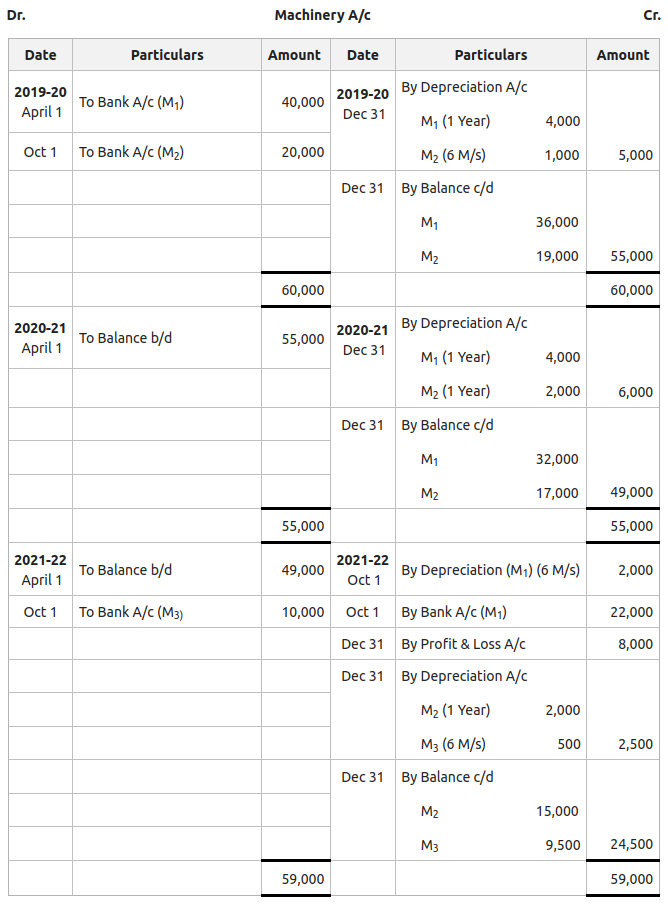My Personal Notes arrow_drop_up
Related Articles